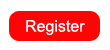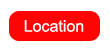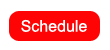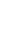FUNCTIONS:

Basic Functions:

• Average (average of a range of cells)
• Max (highest number in a range cells)
• Min (lowest number in a range of cells)
• Sum (sum or total of a range of cells)

Count Functions:

• Count (count cells containing a number)
• CountA (count non-blank cells containing text or a number)
• CountBlank (count blank cells)
• CountIf (count only those cells meeting a single criteria - a word, a number, etc.)
• CountIfs (count only those cells meeting multiple criteria - word, number, etc.)

Sum Functions:

• SumIf (sum or total only those cells meeting a single criteria - a word, a number, etc.)
• SumIfs (sum or total only those cells meeting multiple criteria - words, numbers, etc.)
• SumProduct (sum the product of multiple rows)

If Functions:

• If (check to see if cells meet a single criteria and assign a result - we also incorporate AND & OR conditions)
• And (assign a True or False value to rows that meet multiple criteria)
• Or (assign a True or False value to rows meet one or another criteria)
• IfError (substitute something else if forumal results in an error message)

HLookup & VLookup Functions:

• HLookup (have Excel look up data in a table)
• VLookup (have Excel look up data in a table)

Financial Functions:

• Pmt (calculate payments and interest rates on car and home loans)

Text Functions:

• Concatenate (combine data from multiple fields into one)
• Trim (remove extra blank spaces between Concatenated text)
• Upper (convert all text to uppercase)
• Lower (convert all text to lowercase)
• Proper (convert text to capitalize first letter of each word)
• Left (extract a number of characters from the left side of a text string)
• Right (extract a number of characters from the right side of a text string)
• Mid (extract a number of characters from the middle of a text string)
• Text to Columns (separate text into columns)

Date /Time Functions:

• Date (display current date)
• Now (display current date/time)
• Calculate number of days/months/years between two different dates
• Month
• Year

Misc:

• AverageIf (average a range cells if they meet a single criteria)
• AverageIfs (average a range cells if they meet multiple criteria)
• "Nest" functions (place one function within another)
• Workbook Controls (Sliders, Spin Button, Option Button) to create an interactive spreadsheet
• Dashboard (create one sheet that combines charts, sheets, workbook controls, etc)

GOAL SEEK:

Goal Seek allows you to calculate an unknown value in a given formula, but is only useful for problems that involve finding a single variable. When the desired result of a calculated cell is known, but not the input value that calculation needs to reach that result, you can use Goal Seek.

SOLVER:

Solver is a tool that helps you find solutions involving multiple variables.

SCENARIOS:

Scenarios are part of a group of commands that can be called what-if analysis tools. A scenario is a set of values that a user can create and save and then substitute at any time in the worksheet. The user can then switch to any of these new scenarios to view different results in the worksheet. To compare several scenarios, you can create a report that summarizes them on the same page.

"WHAT-IF" TABLES:

What-If Tables allow you to analyze data and produce a table to show the results. When a single variable like the unit price changes, use a one-input What-If Table. If the unit price AND number of units sold changes, use the two-input What-If Table.

MACROS:

Create Absolute and/or Relative Macros to automate common, repetitive tasks.

WORKBOOK CONTROLS: